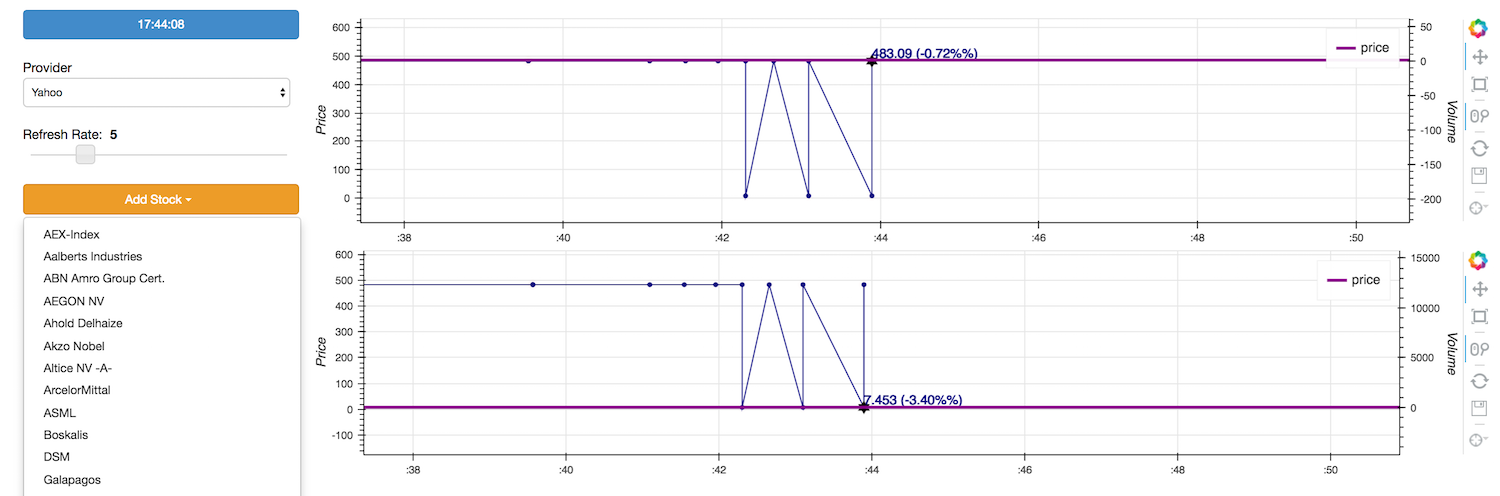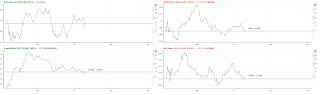# How can I implement live plotting of multiple plots on one single page

My solution for live stock price plotting:

• bokeh sever running

• start page based on MultiPlot class (see below)

• Plot class (see below)

On the start page the user can add a Plot for each stock by selecting it from a list.

Multiplot class has:

• self.div = Div(text = “”, width = 1200, height = 0) where all plots are added

• Dropdown with stock list (to select by the user)

• Plot list for holding added plots = Plot objects, instantiated with: plot = Plot(params)

rootLayout = curdoc().get_model_by_name(self.plot_container)

listOfSubLayouts = rootLayout.children

listOfSubLayouts.append(plot.get_figure())

• init method with:

mainLayout = column(row(self.div, name = “Multiple Plots Container”))

session = push_session(curdoc())

session.show()

session.loop_until_closed()

• update method with:

for iter in self.plots:

``````self.plots[iter].update(stock_data)
``````

Plot class has:

• self.plot_main = Figure()

• source_lines = ColumnDataSource(data_source_dict)

• self.lines = self.plot_main.line(params, source = self.source_lines)

• update method with self.source_lines.stream(new_source_data)

What I am getting are two identical plots (see attached image) with a saw pattern. The points (bullets) match the data. The problem is that the points are not being connected with each other with lines but always go to point 0 there between

Any clue what I am doing wrong?Hi Bryan,

I think I made my question too complicated because no one answers…

I noticed that the plot data is being mixed when having 2 live-plots on the same page and when using stream method to one bokeh server.

Could you confirm that one bokeh server can handle only one live-plot?

Or how many live-plots can a single bokeh server handle?

With one plot all works fine. When I add another plot the drawing looks liek in the screenshot above.

Thanks.

···

On Thursday, January 12, 2017 at 10:10:36 PM UTC+1, [email protected] wrote:

My solution for live stock price plotting:

• bokeh sever running
• start page based on MultiPlot class (see below)
• Plot class (see below)

On the start page the user can add a Plot for each stock by selecting it from a list.

Multiplot class has:

• self.div = Div(text = “”, width = 1200, height = 0) where all plots are added
• Dropdown with stock list (to select by the user)
• Plot list for holding added plots = Plot objects, instantiated with: plot = Plot(params)

rootLayout = curdoc().get_model_by_name(self.plot_container)

listOfSubLayouts = rootLayout.children

listOfSubLayouts.append(plot.get_figure())

• init method with:

mainLayout = column(row(self.div, name = “Multiple Plots Container”))

session = push_session(curdoc())

session.show()

session.loop_until_closed()

• update method with:

for iter in self.plots:

``````  self.plots[iter].update(stock_data)
``````

Plot class has:

• self.plot_main = Figure()
• source_lines = ColumnDataSource(data_source_dict)
• self.lines = self.plot_main.line(params, source = self.source_lines)
• update method with self.source_lines.stream(new_source_data)

What I am getting are two identical plots (see attached image) with a saw pattern. The points (bullets) match the data. The problem is that the points are not being connected with each other with lines but always go to point 0 there between

Any clue what I am doing wrong?I managed to get it work properly by having a unique name for the data_source dictionary per plotexec(“self.source_” + data[‘name’] + " = ColumnDataSource({‘time’: , ‘price’: })")
self.plot_main = figure(title = data[‘name’], x_axis_type = “datetime”)
self.lines = self.plot_main.line(x = ‘time’, y = ‘price’, source = eval(“self.source_” + data[‘name’]))

``

···

On Thursday, January 12, 2017 at 10:10:36 PM UTC+1, Tony Halik wrote:

My solution for live stock price plotting:

• bokeh sever running
• start page based on MultiPlot class (see below)
• Plot class (see below)

On the start page the user can add a Plot for each stock by selecting it from a list.

Multiplot class has:

• self.div = Div(text = “”, width = 1200, height = 0) where all plots are added
• Dropdown with stock list (to select by the user)
• Plot list for holding added plots = Plot objects, instantiated with: plot = Plot(params)

rootLayout = curdoc().get_model_by_name(self.plot_container)

listOfSubLayouts = rootLayout.children

listOfSubLayouts.append(plot.get_figure())

• init method with:

mainLayout = column(row(self.div, name = “Multiple Plots Container”))

session = push_session(curdoc())

session.show()

session.loop_until_closed()

• update method with:

for iter in self.plots:

``````  self.plots[iter].update(stock_data)
``````

Plot class has:

• self.plot_main = Figure()
• source_lines = ColumnDataSource(data_source_dict)
• self.lines = self.plot_main.line(params, source = self.source_lines)
• update method with self.source_lines.stream(new_source_data)

What I am getting are two identical plots (see attached image) with a saw pattern. The points (bullets) match the data. The problem is that the points are not being connected with each other with lines but always go to point 0 there between

Any clue what I am doing wrong?